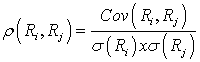Tags
Question
The correlation between two random variables, Ri and Rj, is defined as:

p(Ri,Rj) = [...]Tags
Question
The correlation between two random variables, Ri and Rj, is defined as:

p(Ri,Rj) = [...]
?

Tags
Question
The correlation between two random variables, Ri and Rj, is defined as:

p(Ri,Rj) = [...]If you want to change selection, open document below and click on "Move attachment"

Subject 7. Covariance and Correlation
ame time. We know that this is the case because both returns are higher in favorable conditions and lower in unfavorable conditions. Had we obtained a negative answer, logic would have told us that we had made an error somewhere. <span>The correlation between two random variables, R i and R j , is defined as: Alternative notations are corr(R i , R j ) and ρ ij . Properties of correlation: Correlation is a number between -1 and

#### Summary

status measured difficulty not learned 37% [default] 0

No repetitions